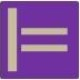## 1 + x = 3 as a Query

Just a brief recap of some software engineering fundamentals:

## FOL Query

What is

(1) 1 + x = 3, with x ∈ N

from a software engineering perspective? Can it be considered a requirement? No, because it’s not a sentence and thus cannot be evaluated to true/ false.
Can it be considered a query? Yes, since it defines a result set on N, that is {(2)}.
This result set contains a single 1-ary tuple. Other cases are:
1 + x <= 3, {(1), (2)}
3 + x = 1, {}
1 + 2 = 3, {()}
3 + 2 = 1, {}

Here "()" is said to be a tuple with arity 0. For example, a 2-tuple (1, 2) can be defined by a set {{1}, {1, 2}}. Thus a 1-tuple (1) is {{1}}, and a 0-tuple () is {}. Thus, {()} and {} correspond to truth values true and false.

## SQL Query

An SQL version would go like this: we define a table “+” (cols y, x, z) that contains all possible results (y+x=z) (what would make it actually infinite, but nerver mind), that we can query by

(2) select x from + where y = 1 and z = 3;

or alternatively for a table N of all naturals (col n) and a query language containing a + operator:

(3) select n from N where 1 + n = 3;

## Boolean Query

Back to First Order Logic. Also the following equation can be considered a query of 0-ary tuples, a so called boolean query:

(4) ∃x 1 + x = 3

Notice, this could also be seen as a requirement, since it evaluates to true/ false. So could “1+2=3”. However, requirements are usually about sets not about single instances, i.e. Boy loves Girl, not Willy loves Kate. So, “1+2=3” would correspond more to a test case.

So long
|=